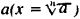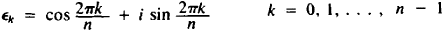Binomial Equation

binomial equation

[bī′nō·mē·əl i′kwā·zhən]
(mathematics)
An equation having the form xn-a = 0.
McGraw-Hill Dictionary of Scientific & Technical Terms, 6E, Copyright © 2003 by The McGraw-Hill Companies, Inc.
The following article is from The Great Soviet Encyclopedia (1979). It might be outdated or ideologically biased.

Binomial Equation

an equation of the form xna = 0, where a is some real or complex number. The solution of these equations is a consequence of the problem of extracting the nth root of the number. The binomial equation has n different roots including no more than two real roots. If a is a positive number, then one of these roots—the arithmetic root—is positive. When geometrically representing numbers in the complex plane, all roots of the binomial equation are located on a circle with the center at point O and a radius equal to the arithmetic root of the modulus of the number a (at the apexes of an n-sided polygon).

Binomial equations of the special form xn − 1 = 0 are of great importance. The roots of these equations are called nth roots of unity and have the formThe product and the quotient of two nth roots of unity will also be the nth roots of unity. Among the roots of the nth roots of unity there are such that all the remaining roots are represented as their powers; these roots are called primitive roots. For the root k to be primitive, it is necessary and sufficient that the numbers k and n be relatively prime numbers, namely, that their greatest common denominator be equal to unity. For example, the root 1, is always primitive: k = 1k.

The theory of binomial equations has made it possible to find the conditions for the solubility of the ancient problem of the division of a circle into equal parts using a compass and a straightedge.

REFERENCES

Okunev, L. la. Vysshaia algebra, 2nd ed. Moscow, 1966.
Kurosh, A. G. Kurs vysshei algebry, 9th ed. Moscow, 1968.